﻿ 中国股市复杂网络中的分形特征文章快速检索 高级检索

Fractal characteristic of the Chinese stock market complex network
ZHUANG Xin-tian, , YUAN Ying, ZHUANG Xiao-wei
School of Business & Management, Northeastern University, Shenyang 110819, China
Abstract:By using the threshold method, this paper constructs the Chinese stock market complex network and analyzes the fractal characteristics of the network from the dimensions of time and space. Firstly, the static network is analyzed with the fractal geometry theory, from which the fractal dimension of the network is obtained. The result shows that the fractal dimension decreases with the threshold. Then, based on the R/S analysis method, this paper analyzes the time series of the clustering coefficient of network. The result illustrates that the time series show the long memory and persistence. And in the long time window, this result is more trustworthy. Approximately, the Hurst index increases when the time window and the threshold increase. The cycle increases when the time window increases and the threshold decreases. It illustrates that in the real market, the trader's group behavior inertia is stronger in the case of long time window and high correlation stocks, and the cycle of the trader's group behavior inertia is longer in the case of long time window and low correlation stocks.
Key words: Chinese stock market     complex network     fractal dimension     R/S analysis     Hurst index

0 引言

 $R_i (t)=\ln (P_i (t)/P_i (t-1))$ (1)

 $C_{ij}=\frac{E(R_{i}R_{j})-E(R_{i})E(R_{j})}{\sqrt{Var(R_{i})Var(R_{j})} }$ (2)

 $\begin{equation} W=\left\{ \begin{array}{ll} w_{ij} =1,& i\ne j\ \mbox{and}\ c_{ij} \ge \theta \\ w_{ij} =0,& i\ne j\ \mbox{and}\ c_{ij} <\theta \\ w_{ij} =0,& i=j \\ \end{array} \right. \end{equation}$ (3)

 $\begin{eqnarray} C_i =2E_i /[k_i (k_i -1)] \end{eqnarray}$ (4)

 $\begin{eqnarray} C(N)\propto N^{-D} \end{eqnarray}$ (5)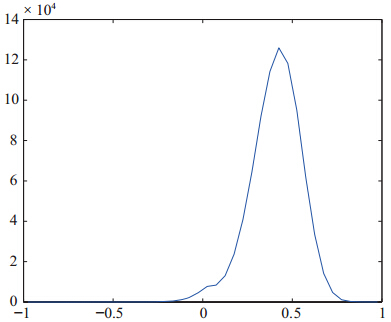图 1 网络相关系数概率分布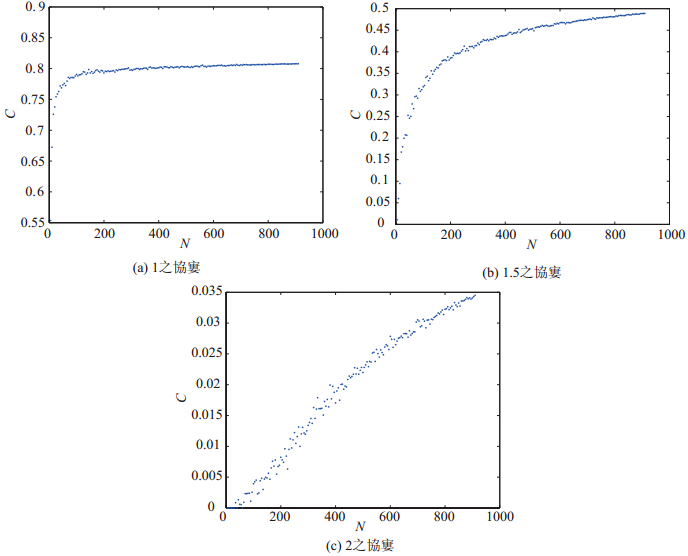图 2 网络的节点数目与聚集系数散点图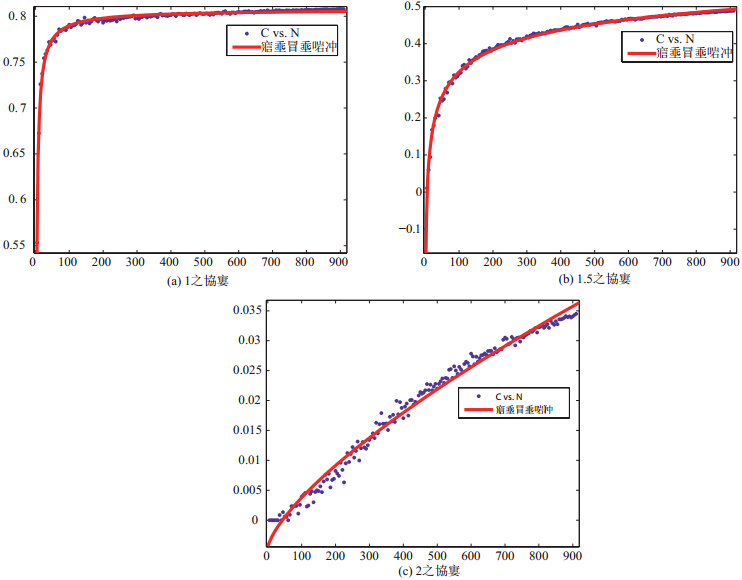图 3 网络的节点数目与聚集系数指数函数拟合图

 $\begin{eqnarray} C(N)=aN^b+c \end{eqnarray}$ (6)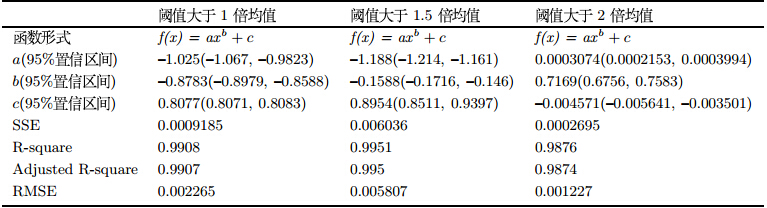1.4 结果分析及讨论

 $\begin{eqnarray} C(N)=-1.025N^{-0.8783}+0.8077 \end{eqnarray}$ (7)

 $\begin{eqnarray} C(N)=-1.188N^{-0.1588}+0.8954 \end{eqnarray}$ (8)
 $\begin{eqnarray} C(N)=0.0003074N^{0.7169}-0.004571 \end{eqnarray}$ (9)

1)从1990年12月19日起选取15天的数据构建一个网络.

2)将时间窗口后移一天,得到第二个网络.

3)再把时间窗口后移一天,直至最后,得到一小族共5083个网络.

4)选取阈值分别为0.4,0.5,0.6,0.7,进行上述步骤,共得到4小族网络.这4小族网络共同组成了1大族网络.

5)将时间窗口分别设为30天,60天,120天重复上述步骤,分别得到3大族网络,12小族网络.其中30天大族网络共4小族,每 小族网络个数为5068;60天大族网络共4小族,每个数网络个数为5038;120天大族网络共4小族,每小族网络个数为4078.

6)共得到4大族,16小族共77068个网络,分别计算聚集系数.可以得到4组,16个基本时间序列.

7)类似于计算收益率时间序列,用$P_{i}(t)$表示时间序列$i$在时期$t$的数值,$R_{i}(t)=\ln(P_{i}(t)/P_{i}(t-1))$定义为时间序列$i$从第($t-1$)期到第$t$期的对数变化率,从而得到16个用于下文计算的变化时间序列.

Hurst提出了R/S分析方法,采用统计量$H$来识别系统性的非随机特征,即Hurst指数,其计算的具体步骤如下:

1)定义长度为$N$的收益序列{\{}$R_{t}${\}},并将它分割为长度为$n$的$A$个连续子区间.将每一子区间标为$I_{a}$,$a=1,2,\cdots,A$.于是$I_{a}$中每一点可以表示为$R_{k,a}$,$k=1,2,\cdots,n$; $a=1,2,\cdots,A$.

2)对每一长度为$n$的子区间$I_{a}$,计算其均值为:
 $e_a =\frac{1}{n}\sum\limits_{k=1}^n {R_{k,a} }$ (10)

 $X_{k,a} =\sum\limits_{i=1}^k {(R_{i,a} -e_a )} ,k=1,2,\cdots,n$ (11)

3) 由式(11)可知,单个子区间累积均值离差序列{\{}$X_{1,a},X_{2,a},\cdots,X_{n,a}${\}}之和为零.定义单个子区间的极差为:
 $R_{I_{a}}=\max(X_{k,a})-\min(X_{k,a}),k=1,2,\cdots,n$ (12)

4)计算每一子区间的标准差$S_{Ia}$,并用它对极差进行重标度/标准化($R_{Ia}/S_{Ia}$):
 $S_{I_a } =\sqrt {\frac{1}{n}\sum\limits_{k=1}^n {(E_{k,a} -e_a )^2} }$ (13)

5)因此,对划分长度$n$,可以计算$A$个子区间平均的重标度极差:
 $(R/S)_n =\frac{1}{A}\sum\limits_{a=1}^A {(R_{I_a } /S_{I_a } )}$ (14)

6)对不同的划分长度(即不同的时间尺度)$n$重复以上计算过程,可得到多个平均重标度极差值.$\log(R/S)$与$\log(n)$存在线性关系:
 $\log(R/S)_{n}=a+H\log(n)$ (15)

7)对$n$和$R/S$进行双对数回归,其斜率就是长程相关的参数,即Hurst指数$H$.因此,可以通过普通最小二乘法得出序列的长程相关参数$H$.

Hurst指数能够区分出分形时间序列,并能够进一步判定该序列是持久性序列还是反持久性序列:当$H=0.5$时,该过程是一个独立同分布过程;当$0.52.3 实证研究 本节首先展示2.1节得到的16个时间序列的基本统计量,如表 2所示. 表 2 时间序列的基本统计量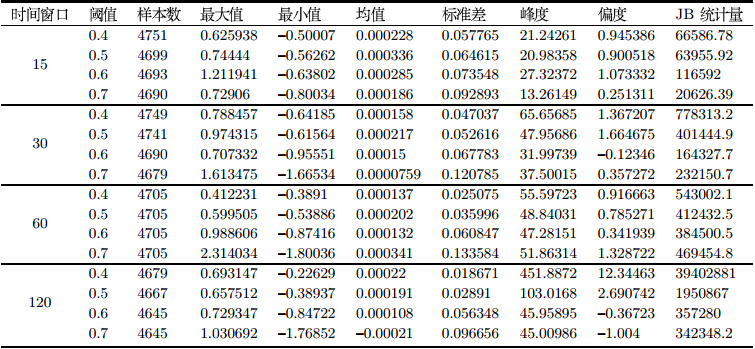表 2可以看出,大部分时间序列呈现右偏,且峰度大于3,表现出尖峰厚尾现象.JB统计量说明时间序列属于非正态分布. 利用R/S分析方法对2.1节得到的16个时间序列进行分析.结果如图 4(只展示部分图片结果)和表 3所示.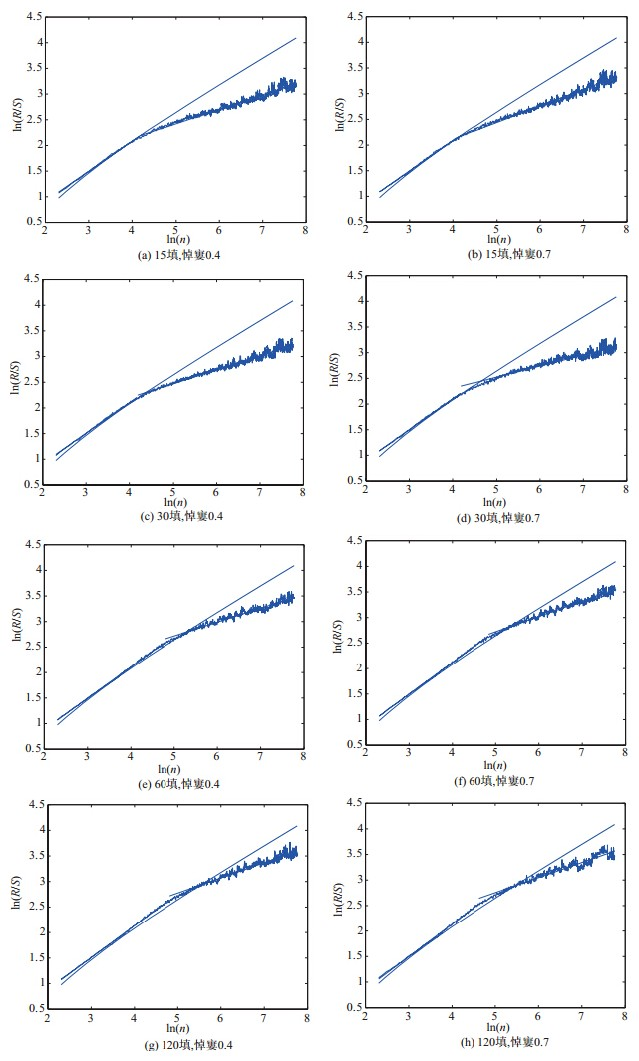图 4 R/S分析结果 表 3 R/S分析结果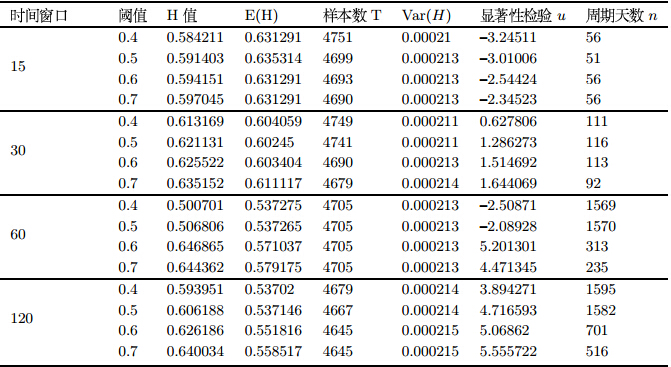其中显著性检验利用公式(16), $u=\frac{H-E(H)}{\sqrt {Var(H)} }\sim N(0,1)$(16) 表 3可以看出,16个时间序列的$H$值均大于0.5,但从显著性检验的结果来看,表中最后6个时间序列通过了$Z_{0.05}=1.96$的显著性检验. 2.4 结果分析及讨论 表 3的结果来看,可以得到以下结论: 1)本节分析的16个时间序列的$H$值均大于0.5,其中通过显著性检验的时间序列有6个,这说明所研究时间序列具有长记忆性和持久性.由2.1节所述,时间序列的实际意义是一种群体交易整体性的变化量,那么这一长记忆性和持久性就可以解释为交易者的群体心理惯性.在实际的市场中,各类投资者虽然交易的时限和频度不同,但是他们都具有自身的某种交易习惯,而且这种交易习惯并不会轻易改变.那么,由交易者引起的市场价格的变化也会形成某种"习惯"且不会轻易改变.因此,由此产生的时间序列就展现出这种长记忆性和持久性,这些性质事实上是交易者群体行为惯性的体现. 2)通过显著性检验的时间序列共有6个,其中60天组有2个,120天组有4个,而其余并未通过检验.这说明这6个时间序列所展示的长记忆性和持久性是值得信赖的,而其余的时间序列的长记忆性和持久性有待进一步确认.考虑到时间序列的实际意义,长时间窗口组别时间序列所包含的交易者群体行为惯性应该较短时间窗口组别的时间序列更为稳定和完整的.这与实际市场中的情况应该是一致的,单个投资者在较长时间形成的交易习惯一般上要比短时间形成的交易习惯更为持久和稳定,而整个市场投资者的群体习惯则更是如此.另外,长时间窗口包含的交易日较多,期间发生的事件和其它因素也较为充分,本身也具备一定的抗扰和去噪的特性.这也使得最终的分析结果更为良好. 3)从$H$值的具体数值来看,大致呈现出随着时间窗口和阈值的增加而增加的规律.也就是说随着时间窗口和阈值的递增,时间序列呈现出的长记忆性和持久性越明显.$H$值随着时间窗口的递增而递增的解释与本小节第二点的结论解释相类似,这里就不再重复了.而$H$值随着阈值的递增而递增则说明了这样的情况.在网络中,高阈值表示的是股票网络整体联系度的增强,那么此时的$H$值较高则说明整体联系度较强的股票网络背后的交易者群体行为惯性也较强.在实际市场中,关联度较高的股票往往是同一板块或是有其它某种共同特征的股票,而相较于低阈值时的情况,投资这些关联度较高股票的投资者的投资范围往往更为集中,对所投资股票的研究程度也较高,所以形成的投资习惯也更难改变,这就使得产生的交易者群体行为惯性更强. 4)从周期天数$n$的数值来看,呈现出随着时间窗口的增加而增长的规律,说明在实际市场中,较长时间窗口下形成的交易者群体行为惯性的循环周期更长,这也应该是得益于长时间窗口形成的交易习惯较为稳定.但在60天和120天组别中,随着阈值的增加,$n$的数值呈下降趋势.这说明在实际市场中,投资关联度较高股票的投资者产生的交易者群体行为惯性的循环周期更短.这是由于在市场中,中国股市的不成熟使得市场股票往往随着宏观及产业政策的变化而出现大面积轮动的现象.这就使得关联度较高的股票种类较低阈值的情况而更不稳定.那么,随着宏观及产业政策的变化,投资于某些关联度较高股票的投资者的投资习惯也会随着股票走势的大面积轮动发生变化,其周期天数必然变小.而在低阈值时,由于此时网络涵盖股票数量较多,受到政策面影响时网络变化不会很大,所以产生的交易者群体行为惯性的持续周期更长. 值得进一步说明的是,$H$值高低表示的是在循环周期内区别于传统的随机游动的趋势性的强度,具体到本节,说明的是投资者在投资关联度较高股票时的交易者群体行为惯性的趋势性的强度,也就是说交易者群体在一定时间内保持这种交易习惯的可能性更大.而循环周期$n$则说明这种趋势性的周期长度,也就是交易者群体保持这种交易习惯的周期.在文献中计算的股票数据中,也存在$H$值和周期天数$n$不一致的现象,但这并不矛盾. 传统分形分析也得到了股市的对记忆性和长程相关性,但是本文与传统方法的研究对象有所不同.传统方法的对象主要是股票指数,而本文是针对复杂网络的宏观参数进行分析,所不同的是,指数事实上是反映了市场股票价格的某种加权平均值,而本文的复杂网络参数是利用了网络的视角得到的整体市场统计特性.相比较而言,以往的分析对象相对简单,而本文的分析方法更贴近市场产生的本源动力,也就是交易者的行为集合. 3 结论 本文从空间和时间两个角度分析中国股市复杂网络的分形特征.在空间角度上,通过建立三个静态网络,利用类似于改变观测尺度的方法计算网络的分形维数.在时间角度上,通过建立中国股市复杂网络聚集系数的时间序列,利用R/S分析方法得出时间序列的分形特征.具体结论如下: 1)按照本文所述的方法,中国股市复杂网络存在空间分形特征,且其分形维数随着网络阈值的增大而减小.这说明,在较高的相关程度下存在相互联系的股票之间的整体相关性较强. 2)在实际市场中,构建投资组合只需要一定的品种数量就可以达到涵盖整体市场相关性的水平. 3)中国股市复杂网络聚集系数时间序列具有长记忆性和持久性,且在长时间窗口下这一性质更值得信赖.从$H$值的具体数值来看,大致呈现出随着时间窗口和阈值的增加而增加的规律.从周期天数$n\$的数值来看,呈现出随着时间窗口的增加而增长,随着阈值的增加而下降的规律.

4)在实际市场中,长时间窗口和高联系度股票产生的交易者群体行为惯性更强,长时间窗口和低联系度股票产生的交易者群体行为惯性的持续周期更长.

  Peters E E. Chaos and order in the capital market[M]. New York: Wiley, 1991: 127-128.  Peters E E. Fractal market analysis[M]. New York: Wiley, 1994: 38-47.  崔鑫,邵芸,王宗军. 资本市场非线性理论研究综述与展望[J]. 管理科学学报, 2004, 7(3): 75-85. Cui Xin, Shao Yun, Wang Zongjun. On non-linearity in capital market: Summary and prospect[J]. Journal of Management Sciences in China, 2004, 7(3): 75-85.  王新宇,宋学峰,吴瑞明. 中国证券市场的分形分析[J]. 管理科学学报, 2004, 7(5): 67-74. Wang Xinyu, Song Xuefeng, Wu Ruiming. Fractal analysis of China stock markets[J]. Journal of Management Sciences in China, 2004, 7(5): 67-74.  宿成建,缪晓波,刘星. 中国证券市场的非线性特征与分形维分析[J]. 系统工程理论与实践, 2005, 25(5): 68-73. Su Chengjian, Miao Xiaobo, Liu Xing. Nonlinear characteristics and fractal dimension analysis of Chinese stock markets[J]. Systems Engineering —— Theory & Practice, 2005, 25(5): 68-73.  都国雄,宁宣熙. 上海证券市场的多重分形特性分析[J]. 系统工程理论与实践, 2007, 27(10): 40-47.Du Guoxiong, Ning Xuanxi. Multifractal analysis on Shanghai stock market[J]. Systems Engineering —— Theory & Practice, 2007, 27(10): 40-47.  魏宇,黄登仕. 基于多标度分形理论的金融风险测度指标研究[J]. 管理科学学报, 2005, 8(4): 50-59. Wei Yu, Huang Dengshi. Study on financial risk measure based on multifractal theory[J]. Journal of Management Sciences in China, 2005, 8(4): 50-59.  周炜星. 上证指数高频数据的多重分形错觉[J]. 管理科学学报, 2010, 13(3): 81-86. Zhou Weixing. Illusionary multifractality in high-frequency data of Shanghai stock exchange composite index[J]. Journal of Management Sciences in China, 2010, 13(3): 81-86.  Grech D, Pamula G. The local Hurst exponent of the financial time series in the vicinity of crashes on the Polish stock exchange market[J]. Physica A, 2008, 387(18): 4299-4308.  Serletis A. Mean reversion in the US stock market[J]. Chaos, Solitons and Fractals, 2009, 40(18): 2007-2015.  Lee C Y. Characteristics of the volatility in the Korea composite stock price index[J]. Physica A, 2009, 388(18): 3837-3850.  Onali E, Goddard J. Are European equity markets efficient? New evidence from fractal analysis[J]. Physica A, 2011, 20(18): 59-67.  苑莹,庄新田. 中国股票市场的长记忆性与市场发展状态[J]. 数理统计与管理, 2008, 27(1): 156-163.Yuan Ying, Zhuang Xintian. Long memory and state of market development in Chinese stock markets[J]. Application of Statistics and Management, 2008, 27(1): 156-163.  Albert R, Barabási A L. Statistical mechanics of complex networks[J]. Reviews of Modern Physics, 2002, 74(1): 47-97.  Newman M E J. The structure and function of complex networks[J]. SIAM Review, 2003, 45(3): 167-256.  刘涛,陈忠,陈晓荣. 复杂网络理论及其应用研究概述[J]. 系统工程, 2005, 23(6): 1-7. Liu Tao, Chen Zhong, Chen Xiaorong. A brief review of complex networks and its application[J]. Systems Engineering, 2005, 23(6): 1-7.  Namaki A, Shirazi A H, Raei R, et al. Network analysis of a financial market based on genuine correlation and threshold method[J]. Physica A, 2011, 390(1): 3835-3841.  Tabak M B, Serra R T, Cajueiro O D. Topological properties of stock market networks: The case of Brazil[J]. Physica A, 2010, 389(2): 3240-3249.  Galazka M. Characteristics of the Polish stock market correlations[J]. International Review of Financial Analysis, 2011, 20(1): 1-5.  李英,曹宏铎,邢浩克. 基于复杂网络少数者博弈模型的金融市场仿真研究[J]. 系统工程理论及实践, 2012, 32(9): 1882-1890.Li Ying, Cao Hongduo, Xing Haoke. Modeling and simulation of complex finance network based on minority game[J]. Systems Engineering —— Theory & Practice, 2012, 32(9): 1882-1890.  蔡世民,洪磊,傅忠谦,等.基于复杂网络的金融市场网络结构实证研究[J].复杂系统与复杂性科学, 2011, 8(3): 29-33. Cai Shimin, Hong Lei, Fu Zhongqian, et al. Empirical study on network structure of financial market based on complex network theory[J]. Complex Systems and Complexity Science, 2011, 8(3): 29-33.  Mandelbrot B B. How long is the coast of Britain? Statistical self-similarity and fractal dimension[J]. Science, 1967, 156(3775): 636-638.
0

#### 文章信息

ZHUANG Xin-tian, ZHANG Ding, YUAN Ying, ZHUANG Xiao-wei

Fractal characteristic of the Chinese stock market complex network

Systems Engineering - Theory & practice, 2015, 35(2): 273-282.